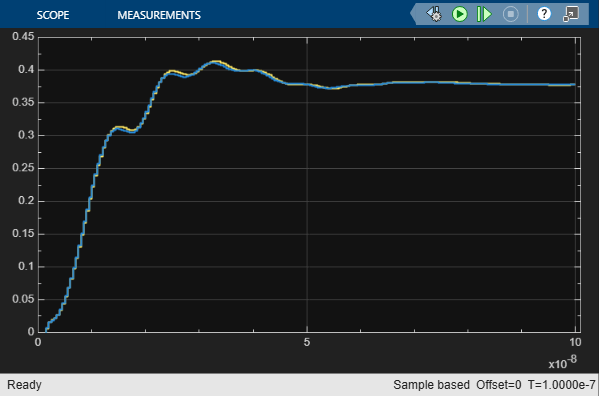Documentation

## Compare Time and Frequency Domain Simulation Options for S-parameters

This example shows how to use two different options for modeling S-parameters with the RF Blockset™ Circuit Envelope library. The Time-domain (rationalfit) technique creates an analytical rational model that approximates the whole range of the data. This is a preferable technique when a good fit could be achieved with a small number of poles. When the data has a lot of details or high level of noise, this model becomes large and slow to simulate.

The frequency-domain technique is based on convolution, where the baseband impulse response depends on the simulation time step and the carrier frequency.

### System Architecture

The system consists of:

• An input envelope signal modeled with Simulink blocks. The input signal is a ramp that goes from 0 to 1 in `TF_RAMP_TIME`; the initial value of `TF_RAMP_TIME` is set to `1e-6` s. The carrier frequency of the signal is `TF_FREQ`; the initial value of `TF_FREQ` is set to `2.4e9` Hz.

• Two SAW filters, modeled by two S-parameter blocks using the same data file, `sawfilter.s2p`. The block labeled `SAW Filter (time domain)` has its Modeling options parameter in the Modeling tab set to `Time domain (rationalfit)`. The block labeled `SAW Filter (frequency domain)` has its Modeling options parameter in the Modeling tab set to `Frequency domain` and the Automatically estimate impulse response duration is checked.

• A Scope block that displays the outputs of the two S-parameter blocks.

```model = 'simrfV2_sparam_t_vs_f'; open_system(model); ```### Run Simulation with the Default Settings

1. Type `open_system('simrfV2_sparam_t_vs_f')` at the Command Window prompt.

2. Select Simulation > Run.

The outputs from both methods are very close to each other. The frequency-domain model (purple curve) captures the transfer function (steady-state value) a bit better.

```scope = [model '/Scope']; open_system(scope); set_param(scope, 'YMax','0.45'); set_param(scope, 'YMin','0'); set_param(scope, 'TimeRange',num2str(1.01*TF_END_TIME)); sim(model); ```### Run the Simulation with the Very Steep Ramp

In the previous simulation, the rise time of the envelope `TF_RAMP_TIME = 1e-6` was many orders of magnitude greater than the period of the carrier signal `T = 1/TF_FREQ = 4.1667e-10`. In other words, the envelope was much slower than the carrier. As the ramp time approaches the period of the carrier, the corresponding time effects are better captured by the time-domain model (yellow curve).

To continue the example:

1. Type `TF_RAMP_TIME = 1e-9; TF_END_TIME = 1e-7;` at the Command Window prompt.

2. Select Simulation > Run.

```TF_RAMP_TIME = 1e-9; TF_END_TIME = 1e-7; set_param(scope, 'TimeRange',num2str(1.01*TF_END_TIME)); sim(model); open_system(scope); ```The result of the frequency-domain simulation can be improved by decreasing the time step of the simulation and manually setting the impulse duration time.

To continue the example:

1. Type `TF_STEP = 5e-10;` at the Command Window prompt.

2. Uncheck Automatically estimate impulse response duration in the modeling pane of `Saw filter (frequency domain)` block and specify the Impulse Response Duration as `1e-7`.

3. Select Simulation > Run.

```TF_STEP = 5e-10; sparam_freq = [model '/SAW Filter (frequency domain)']; set_param(sparam_freq, 'AutoImpulseLength', 'off'); set_param(sparam_freq, 'ImpulseLength', '1e-7'); sim(model); open_system(scope); ```### Run Simulation with Different Frequency

Rational-function approximation is not exact. To see the approximation error, double-click the "SAW Filter (time domain)" block. Information about the approximation appears under "Rational fitting results" in the bottom of the dialog 'Modeling' pane.

```open_system([model sprintf('/SAW Filter (time domain)')]); ```

For more details, select 'Visualization' panel, and click the 'Plot' button.

The rationalfit algorithm (dotted curve) does a very good job for the most of the frequencies. However, sometimes it does not capture the sharp changes of S-parameter data.

```simrfV2_click_dialog_button('Block Parameters: SAW Filter (time domain)', 'PlotButton'); ```Conversely, the frequency-domain method exactly reproduces the steady-state behavior at all carrier frequencies (by definition). Running the simulation for `TF_FREQ = 2.54e9` produces drastically different results between the two S-parameter methods.

To continue the example:

1. Type `TF_FREQ = 2.54e9; TF_RAMP_TIME = 1e-6; TF_STEP = 3e-9; TF_END_TIME = 2.5e-6;` at the Command Window prompt.

2. Select Simulation > Run.

In this case, the frequency-domain model provides a better approximation of the original data.

```TF_STEP = 3e-9; TF_RAMP_TIME = 1e-6; TF_FREQ = 2.54e9; TF_END_TIME = 2.5e-6; set_param(scope, 'YMax','6e-3'); set_param(scope, 'TimeRange',num2str(1.01*TF_END_TIME)); sim(model); open_system(scope); ```### Run Simulation with Impulse Duration Set to Zero.

There is a special case that could be very helpful in practice. When the "Impulse Response Duration" of the s-parameters block is set to zero, the history of the input is no longer taken into consideration. Still, the model captures the transfer function (steady-state value) correctly. This is a fast and reliable way to model the ideal devices when the transient effects could be ignored.

To continue the example:

1. Specify the `Impulse Response Duration` of `Saw filter (frequency domain)` block as `0`.

2. Select Simulation > Run.

```set_param(sparam_freq, 'ImpulseLength', '0'); sim(model); open_system(scope); ```### Conclusion

In most practical RF systems, time- and frequency-domain techniques give similar answers. The time-domain method better captures the time-domain effects of the fast-changing envelopes, but relies on a rationalfit approximation of the original data. The frequency-domain method is sensitive to the simulation time step; this option is recommended when the time-domain model does not provide a good fit.

```close gcf; bdclose(model); clear model scope; ```